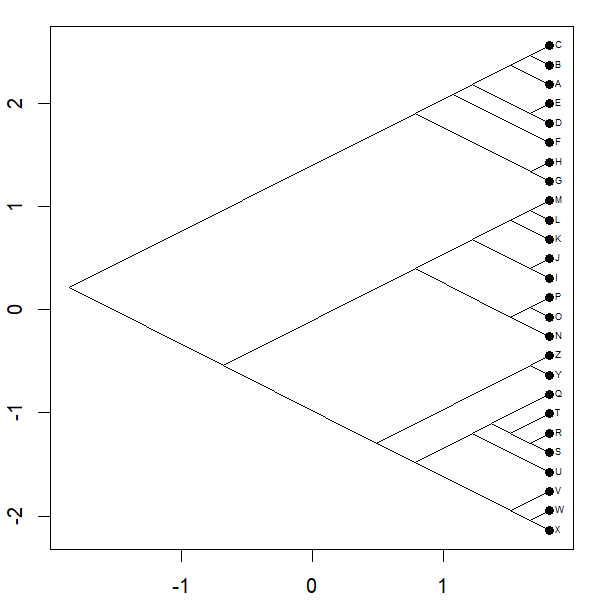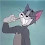## Wednesday, March 14, 2018

### Animated phylomorphospace projection

The so-called 'phylomorphospace' is a projection of the tree into phenotypic space for quantitative traits. Consequently, I thought it might be fun to try & animate this projection - that is, to visualize the transformation of the tree from the way it's typically projected into a phylomorphospace.

This could look something like the following:

``````project.phylomorphospace<-function(tree,X,nsteps=1000,sleep=0,...){
X<-X[tree\$tip.label,]
A<-cbind(fastAnc(tree,X[,1]),fastAnc(tree,X[,2]))
mar<-par()\$mar
obj<-get("last_plot.phylo",envir=.PlotPhyloEnv)
obj\$xx<-obj\$xx*(max(c(X[,1],A[,1]))-min(c(X[,1],A[,1])))+
min(c(X[,1],A[,1]))
xlim<-range(obj\$xx)
xlim<-xlim+max(strwidth(tree\$tip.label))
obj\$yy<-(obj\$yy-min(obj\$yy))/(max(obj\$yy)-min(obj\$yy))*
(max(c(X[,2],A[,2]))-min(c(X[,2],A[,2])))+min(c(X[,2],A[,2]))
ylim<-range(obj\$yy)
X0<-cbind(obj\$xx[1:Ntip(tree)],obj\$yy[1:Ntip(tree)])
rownames(X0)<-tree\$tip.label
A0<-cbind(obj\$xx[1:tree\$Nnode+Ntip(tree)],
obj\$yy[1:tree\$Nnode+Ntip(tree)])
rownames(A0)<-1:tree\$Nnode+Ntip(tree)
par(mar=mar)
phylomorphospace(tree,X0,A0,label="horizontal",xlim=xlim,
ylim=ylim,...)
for(i in 1:nsteps){
Sys.sleep(sleep)
dev.hold()
phylomorphospace(tree,((nsteps-i)*X0+i*X)/nsteps,
((nsteps-i)*A0+i*A)/nsteps,xlim=xlim,ylim=ylim,
...)
dev.flush()
}
}
``````

And if we try it out:

``````library(phytools)
project.phylomorphospace(tree,X,xlab="",ylab="",
node.size=c(0,1.2))
``````Kinda neat?

The .gif I generated as follows:

``````png(file="ppm-%04d.png",width=600,height=600,res=120)
par(mar=c(2.1,2.1,1.1,1.1))
project.phylomorphospace(tree,X,xlab="",ylab="",
node.size=c(0,1),lwd=1,nsteps=100,fsize=0.6)
dev.off()
system("ImageMagick convert -delay 10 -loop 2 *.png 14Mar18c-post.gif")
file.remove(list.files(pattern=".png"))
``````

Finally, here's how I simulated the tree & data:

``````tree<-pbtree(n=26,tip.label=LETTERS)
vcv<-matrix(c(1,-0.8,-0.8,1),2,2)
X<-sim.corrs(tree,vcv)
``````

1.2.